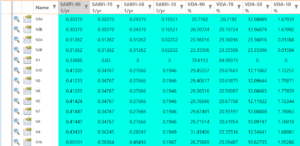# NEPLAN | Voltage Sags

back to overview

The module Voltage Sag Analysis calculates the frequency of critical voltage sags (voltage dips) due to short circuits in the network. Faults are simulated at numerous points of the network, calculating the remaining (retained) voltage of all busbars. This method is known as the method of fault positions.

The results SARFI-90, SARFI-70, SARFI-50 and SARFI-10 are calculated for each node. The SARFI-X values are defined in the IEEE Std 1564-2014 IEEE Guide for Voltage Sag Indices. They indicate how often the voltage falls below a certain limit (X). The unit of SARFI-X is 1/yr. E.g. SARFI-50 indicates how often the voltage falls below 50% of the nominal voltage per year. For each index SARFI-X, the associated value VDA (Voltage Dip Amplitude) is also calculated. VDA is the expected value of the residual voltage during the short circuit and is given as a percentage of the nominal voltage.

### Results

The results are displayed on the single line diagram and in tables:Brochure: Introductory brochure for Voltage Sag calculation could be found here.